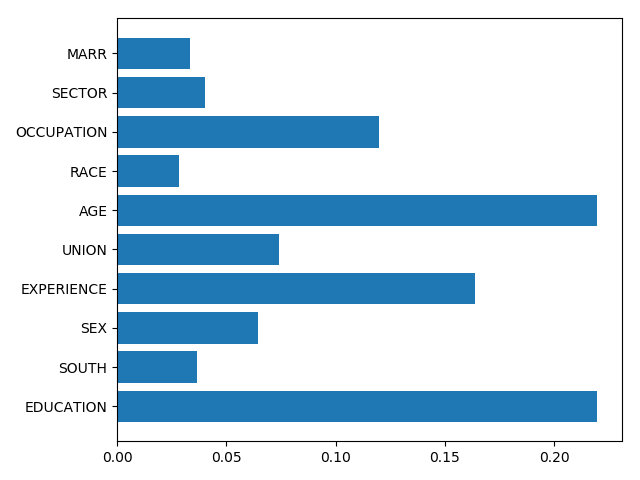# 2.2. Interpreting random forests¶

Interpreting random forests

## 2.2.1. Data on wages¶

We use the same data as in the linear-model notebook

```import os
import pandas

# Python 2 vs Python 3:
try:
from urllib.request import urlretrieve
except ImportError:
from urllib import urlretrieve

if not os.path.exists('wages.txt'):
urlretrieve('http://lib.stat.cmu.edu/datasets/CPS_85_Wages',
'wages.txt')

# Give names to the columns
names = [
'EDUCATION: Number of years of education',
'SOUTH: 1=Person lives in South, 0=Person lives elsewhere',
'SEX: 1=Female, 0=Male',
'EXPERIENCE: Number of years of work experience',
'UNION: 1=Union member, 0=Not union member',
'WAGE: Wage (dollars per hour)',
'AGE: years',
'RACE: 1=Other, 2=Hispanic, 3=White',
'OCCUPATION: 1=Management, 2=Sales, 3=Clerical, 4=Service, 5=Professional, 6=Other',
'SECTOR: 0=Other, 1=Manufacturing, 2=Construction',
'MARR: 0=Unmarried,  1=Married',
]

data = pandas.read_csv('wages.txt', skiprows=27, skipfooter=6, sep=None,
short_names = [n.split(':') for n in names]
data.columns = short_names

# Log-transform the wages, as they typically increase with
# multiplicative factors
import numpy as np
data['WAGE'] = np.log10(data['WAGE'])

features = [c for c in data.columns if c != 'WAGE']
X = data[features]
y = data['WAGE']
```

## 2.2.2. Feature importance¶

```from sklearn import ensemble

forest = ensemble.RandomForestRegressor()
forest.fit(X, y)

# Visualize the feature importance
importance = forest.feature_importances_
from matplotlib import pyplot as plt
plt.barh(np.arange(importance.size), importance)
plt.yticks(np.arange(importance.size), features)
plt.tight_layout()
```## 2.2.3. Meaning and Caveats¶

Feature importances are a proxy for the mutual information between the feature and the target, conditionally on the other features. The conditioning is difficult and not well controlled.

Higher-cardinality categorical variables will have larger feature importances

Total running time of the script: ( 0 minutes 0.086 seconds)

Gallery generated by Sphinx-Gallery